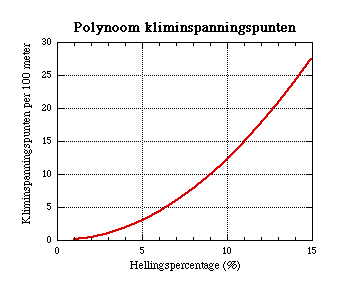# CyclingUp: method

You are visitor nr.The elevation profiles of the ascents are measured with a bike computer, which collects data on the distance covered and on elevation. The profiles are then divided into sections with a length of 250 meters along the road (dL). From the initial and final elevation of each segment the slope (s) is determined and from that the climb effort (dI):

dI = 0.001 s² dL

where dI is in climb effort points (cep), s in % and dL in meters. The total climb effort of the ascent is the sum of the effort points of all sections. This quantity represents only that part of the effort that is spent in addition to the effort spent on a horizontal road. So, the profile is divided into intervals, the climb effort for each interval equals to the number of elevation meters times the slope and the total effort is the sum of the efforts of all intervals. Finally, the result is multiplied by a constant (0.001) so that each elevation meter gained on a slope of 10% results in 1.0 cep. Another elevation meter gained on a slope of 5% adds only 0.5 cep and on a slope of 1% merely 0.1 cep . For details I refer to the Dutch version of this webpage.

Tour for Life
Rijdt deze zomer de unieke en klassieke 8-daagse wielrentocht van Noord-Italië naar Zuid-Nederland voor Artsen zonder Grenzen!